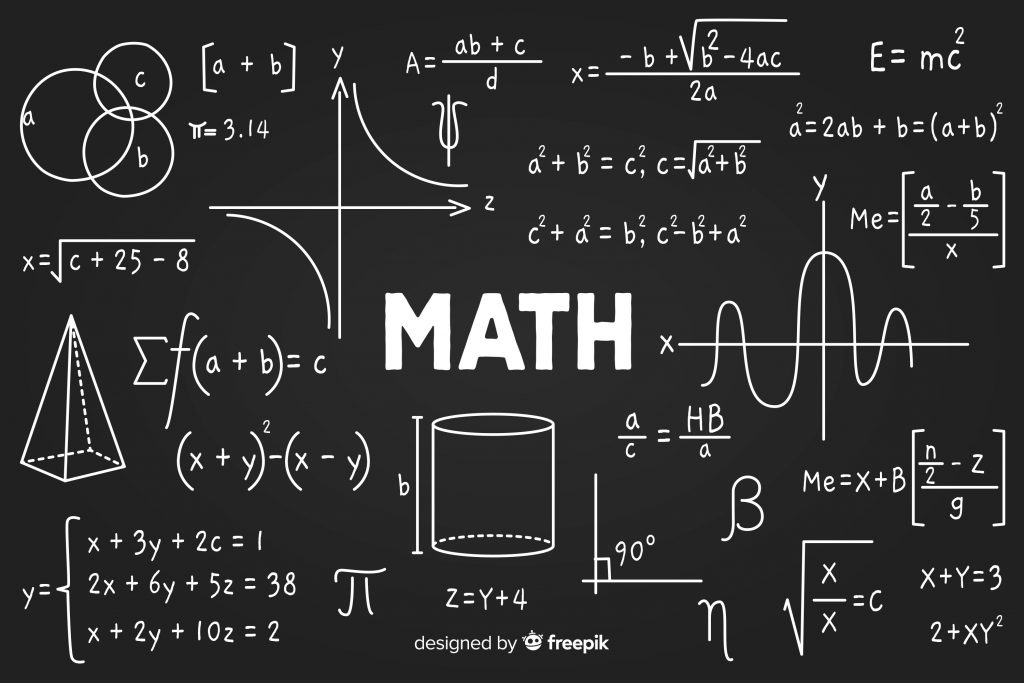5 ways to remember Maths formulas5 ways to remember Maths formulas5 ways to remember Maths formulas5 ways to remember Maths formulas5 ways to remember Maths formulas5 ways to remember Maths formulas5 ways to remember Maths formulas5 ways to remember Maths formulas5 ways to remember Maths formulas